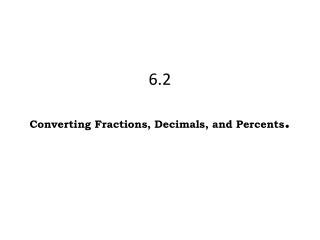DownloadDownload Presentation6.2 Converting Fractions , Decimals , and Percents .

# 6.2 Converting Fractions , Decimals , and Percents .

Télécharger la présentation## 6.2 Converting Fractions , Decimals , and Percents .

- - - - - - - - - - - - - - - - - - - - - - - - - - - E N D - - - - - - - - - - - - - - - - - - - - - - - - - - -
##### Presentation Transcript

1. 6.2Converting Fractions, Decimals, and Percents.

2. 6.2 Directions: Fraction to decimal- Divide the numerator by the denominator Decimal to percent- Multiply by 100. Move decimal 2 places right. Percent to fraction -Percent is the numerator, 100 is denominator then simplify. Percent to decimal- divide by 100. Move decimal 2 places left. Decimal to fraction- Use place value ._ _ _ (tenths, hundredths, thousandths) SAY IT! WRITE IT! (last place value is denominator Foldable FRACTION DECIMAL PERCENT ¼ .25 25% ½ .5 50% ¾ .75 75% 4/4 1 100% 1/5 .2 20% 2/5 .4 40% 3/5 .6 60% 4/5 .8 80% 5/5 1 100% 1/8 .125 12.5% 2/8= ¼ .25 25%

3. Print this worksheet then close this window Pg 10 Pg 9 Page 15

4. pg 12 Ordering Fractions, Decimals, and Percents 1. Convert F, D, and P to the same thing (either all decimal or all percent) Order as asked in question How do you determine the F. D, or P of a shaded shape? You must divide it up yourself to determine the fraction shaded and then convert to decimal or percent as specified. Ex- What is the percentage shaded? ¼ is shaded. ¼ is .25 or 25% Comparing Fractions <, >, =, ≤, ≥ An old trick is to cross multiply. Or you can just covert to a decimal or a percent with a calculator. 5 8 7 8 2 3 33 2 66